# NCERT Solutions for Class 10 Science Chapter 10

NCERT Solutions for Class 10 Science Chapter 10 – Light – Reflection and Refraction, contains solutions to various questions in Exercises for Chapter 10. Light – Reflection and Refraction Class 10 NCERT Solutions have been explained in a simple and easy to understand manner. We are providing all subjects which can be accessed by clicking here.

## Download NCERT Solutions for Class 10 Science Chapter 10 – Light – Reflection and Refraction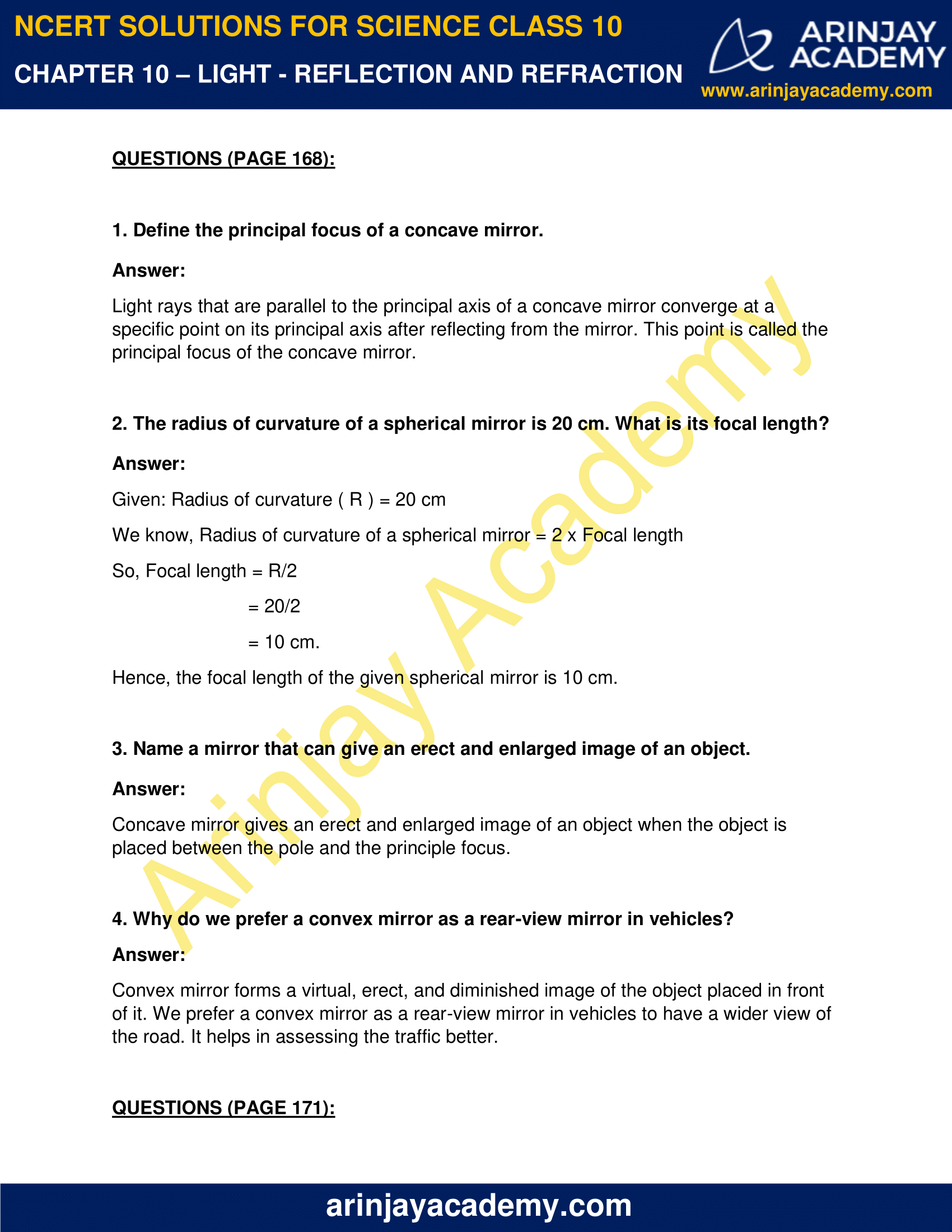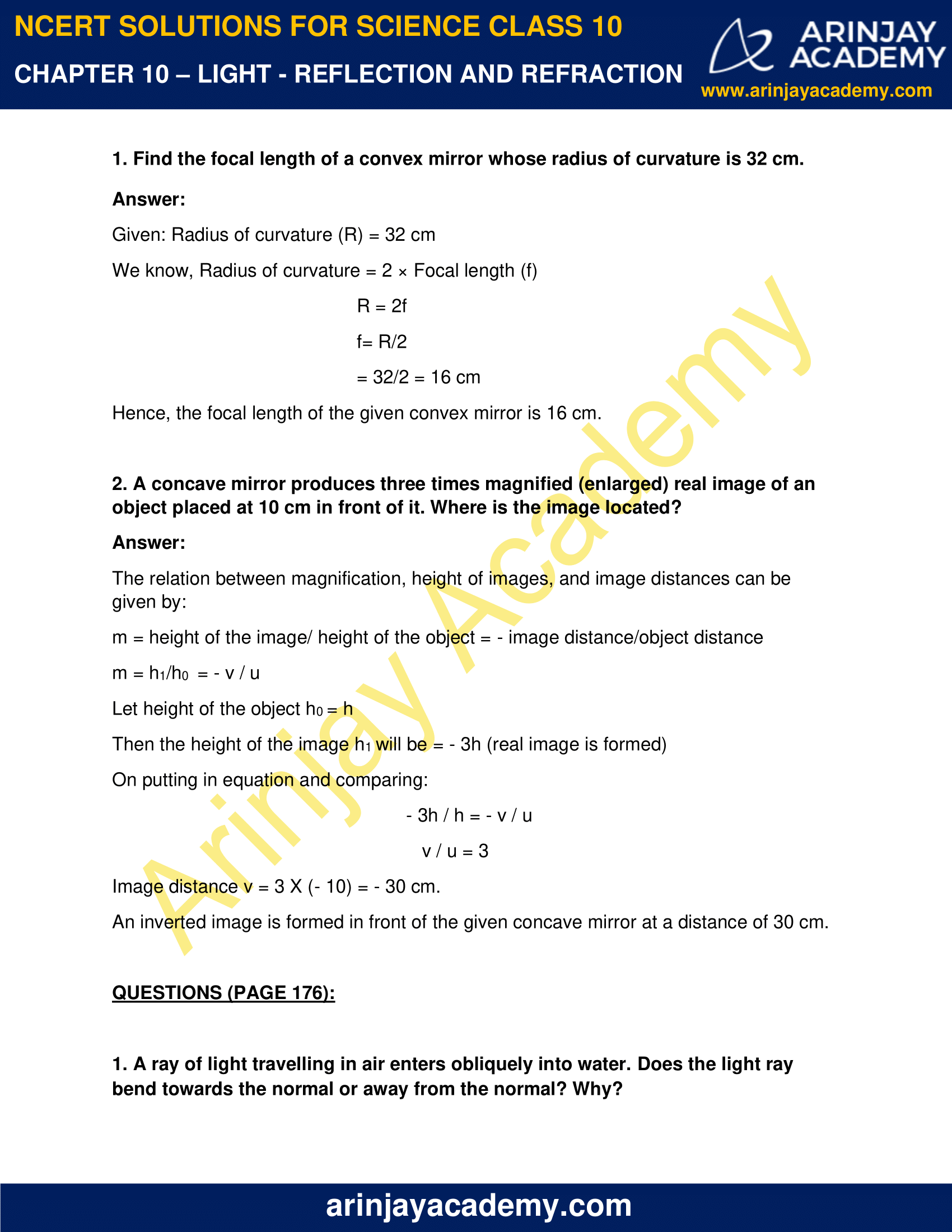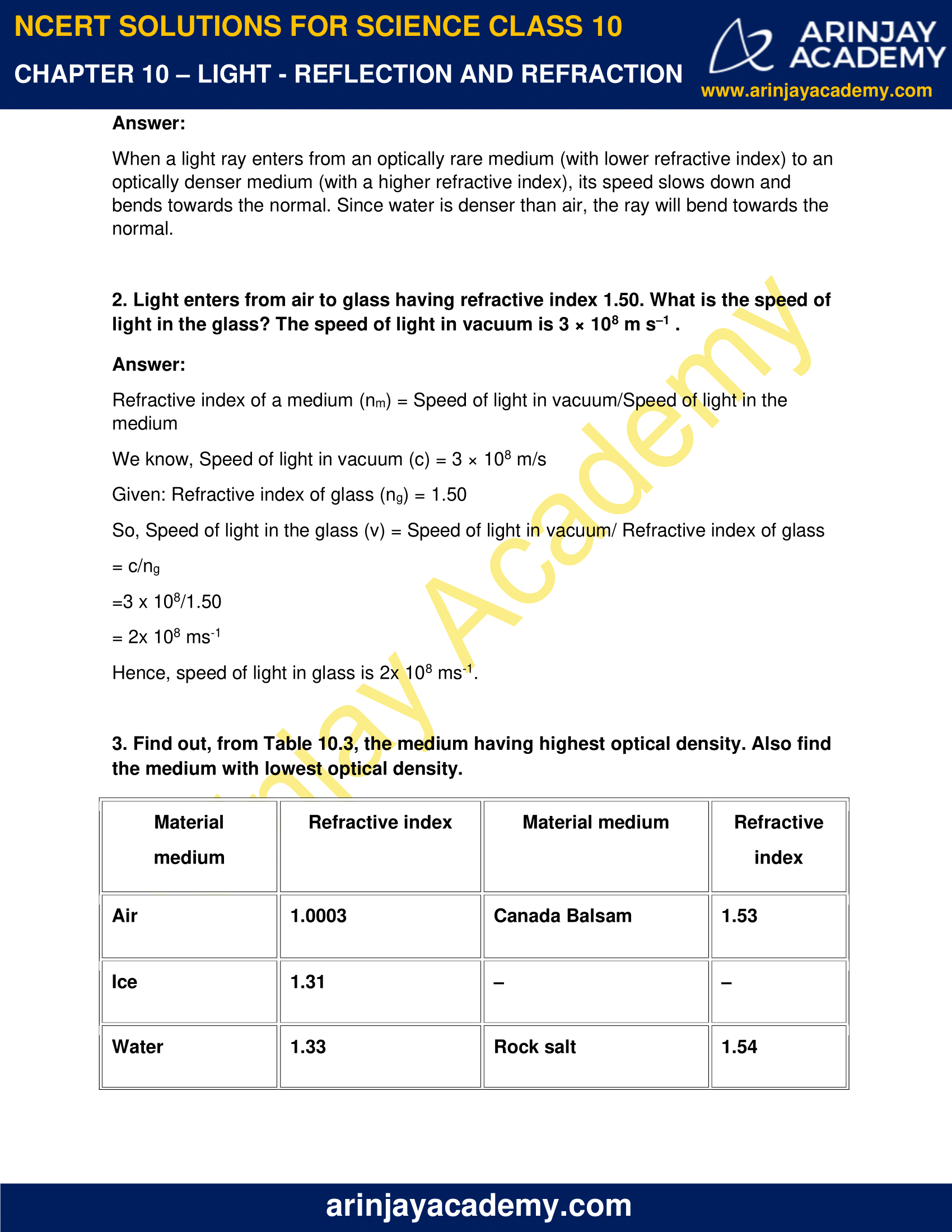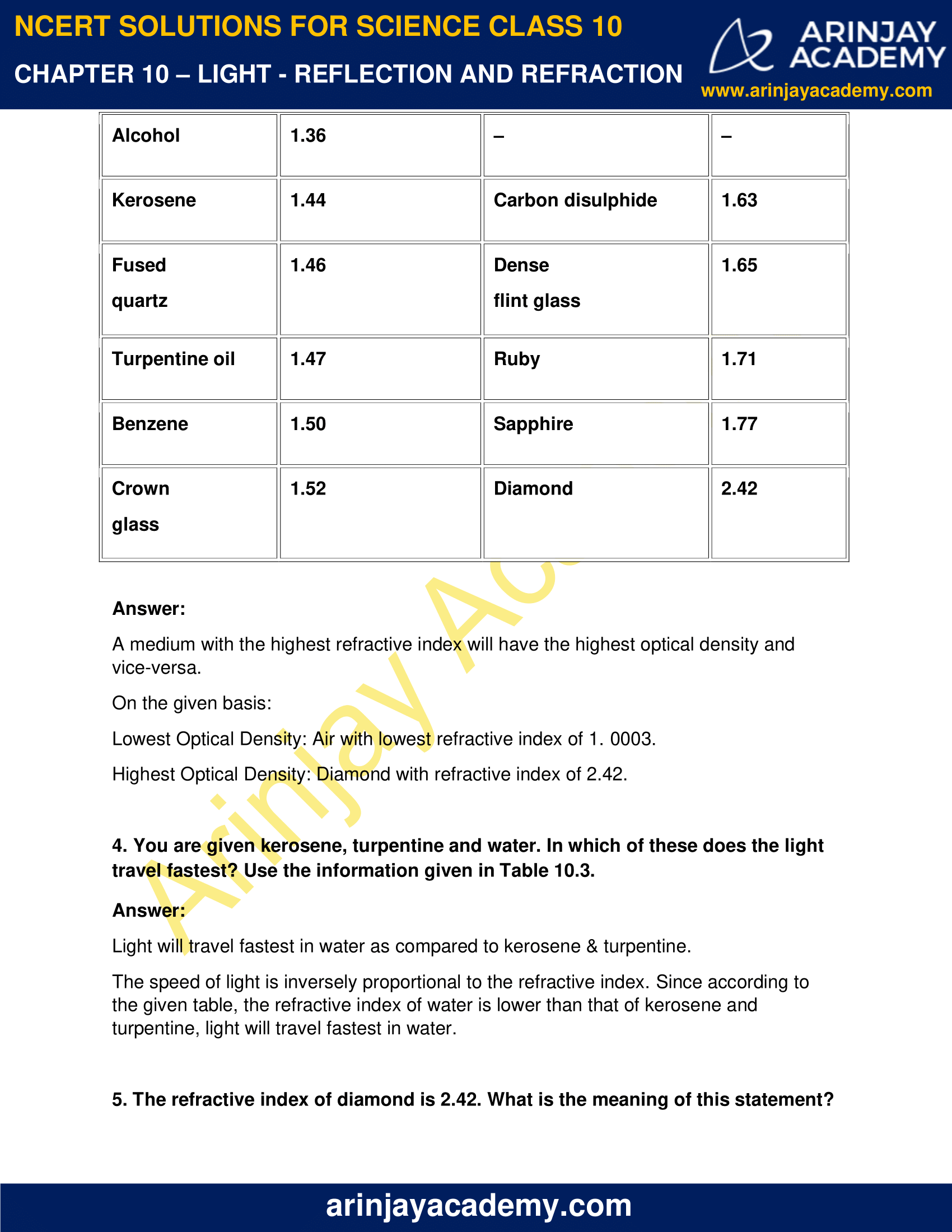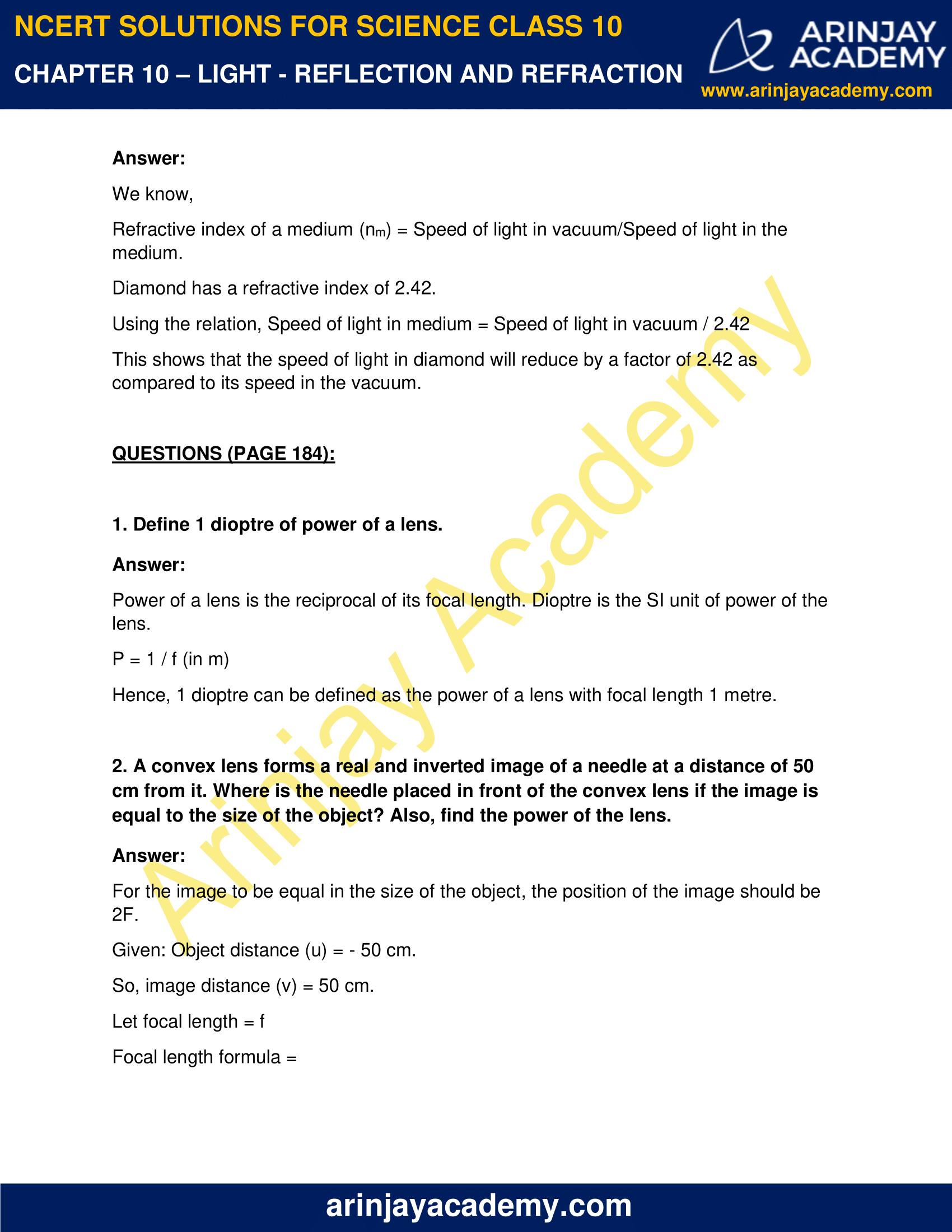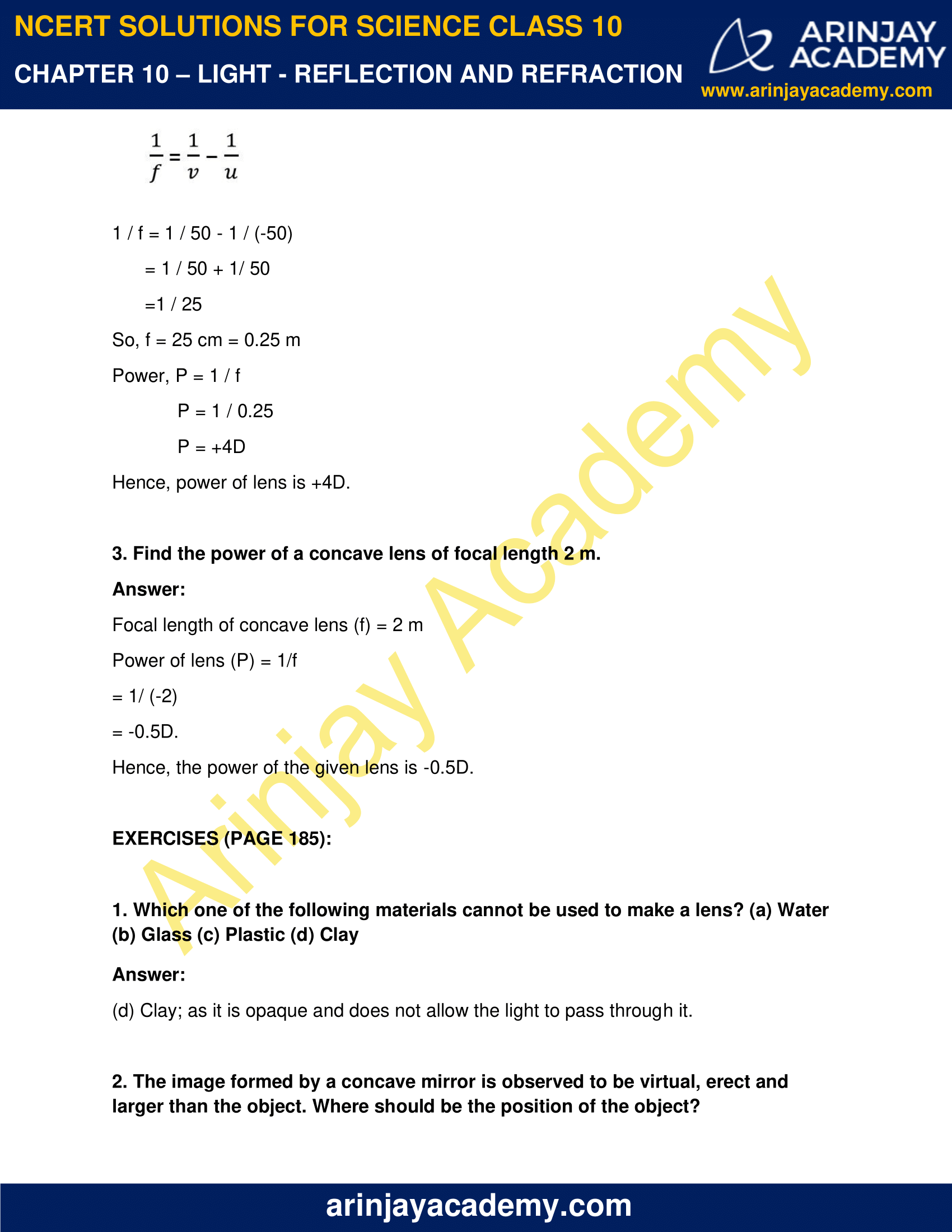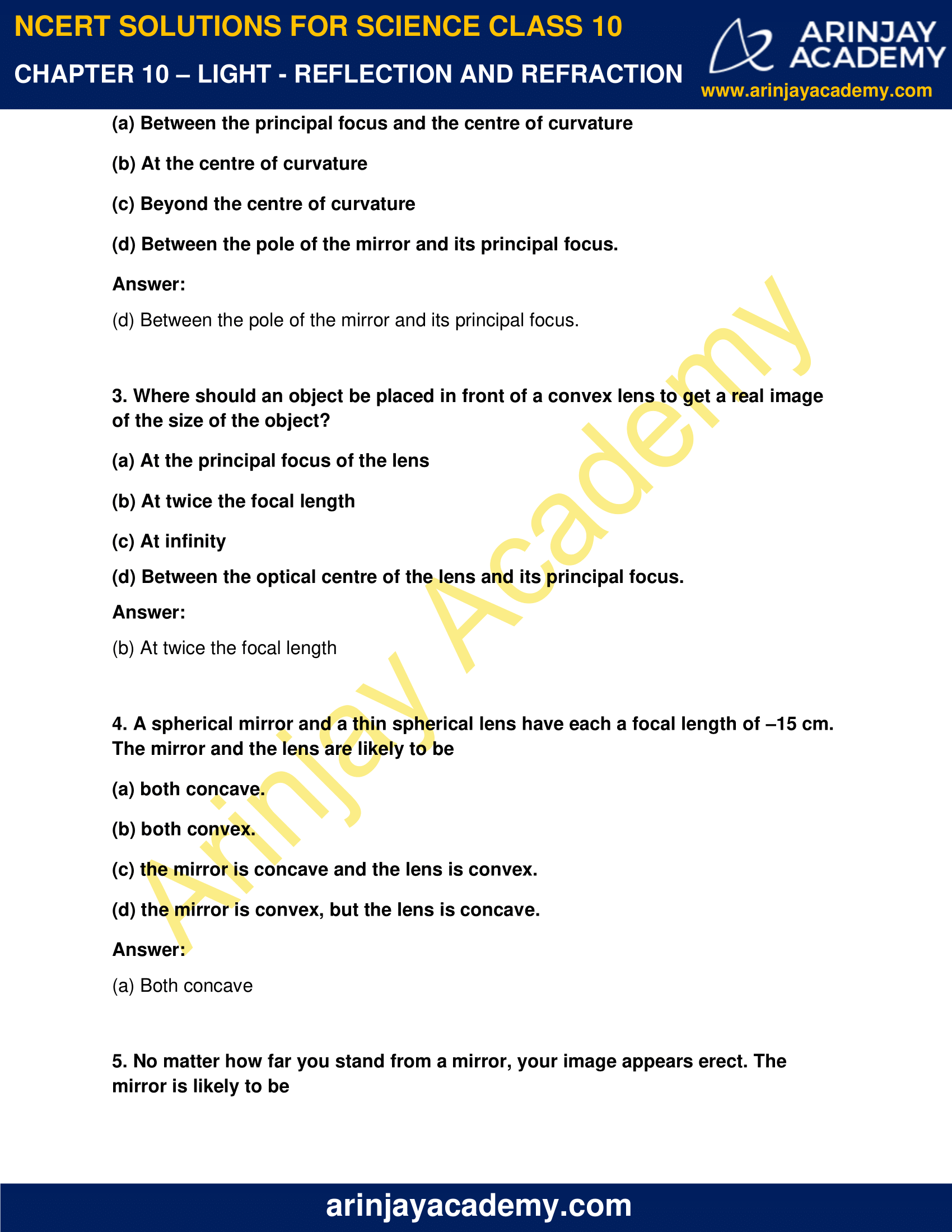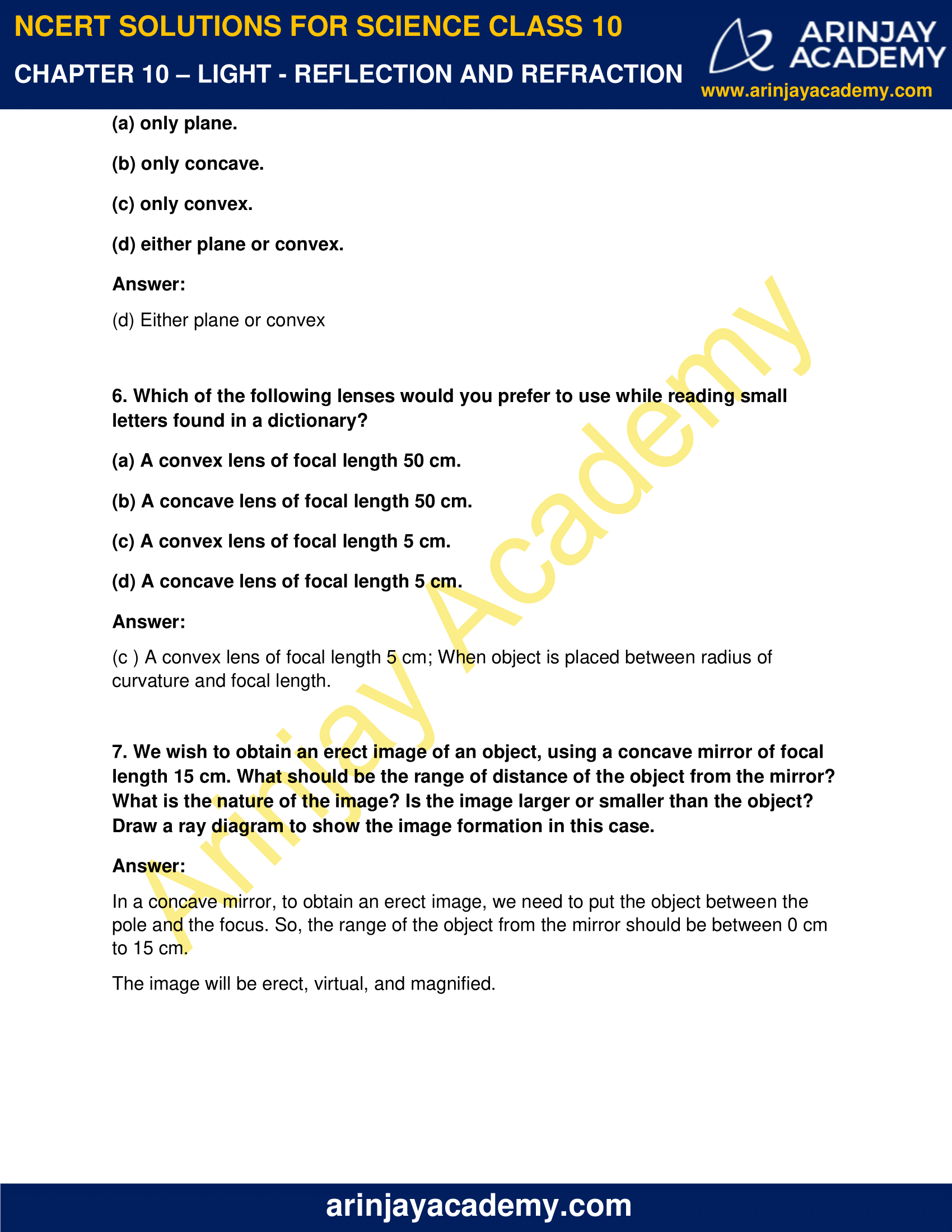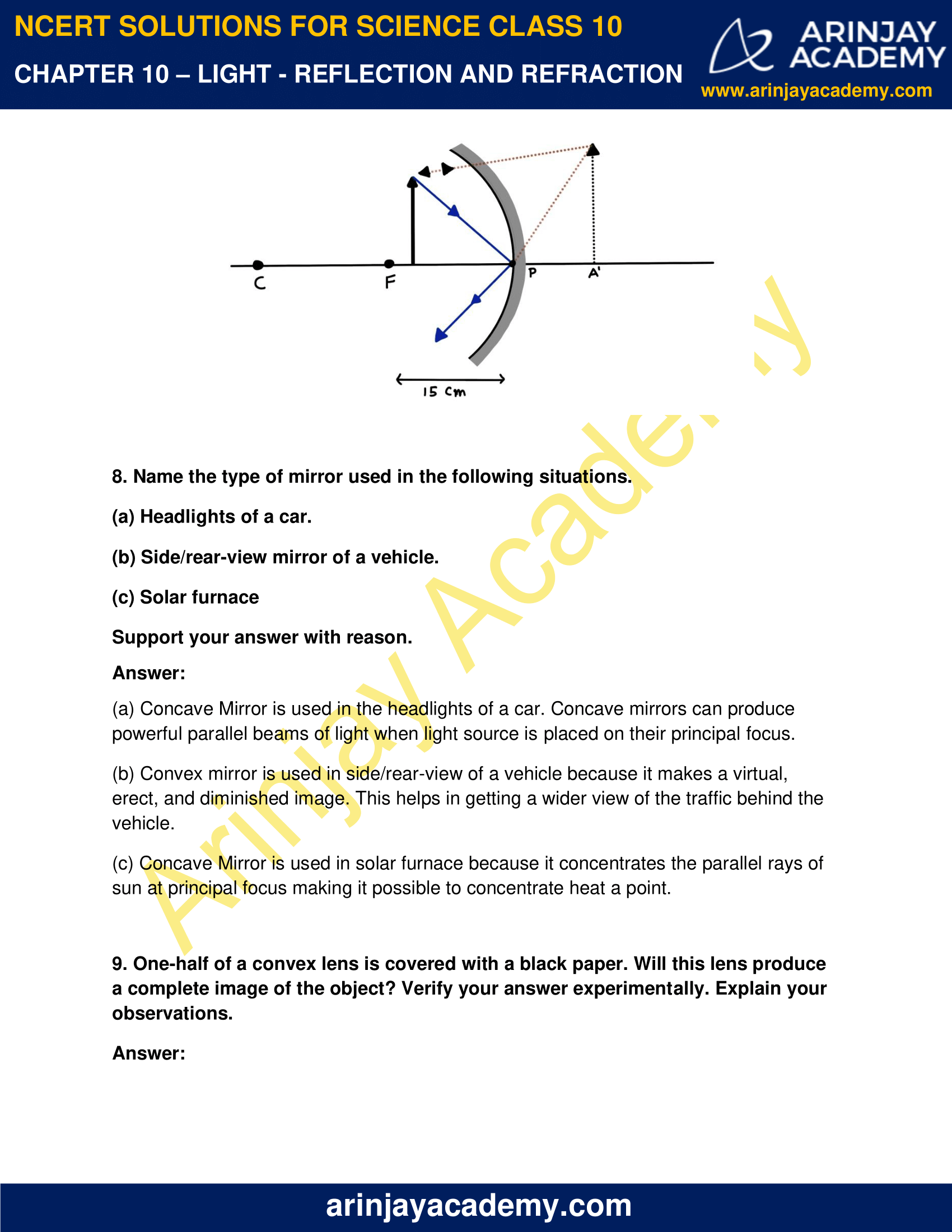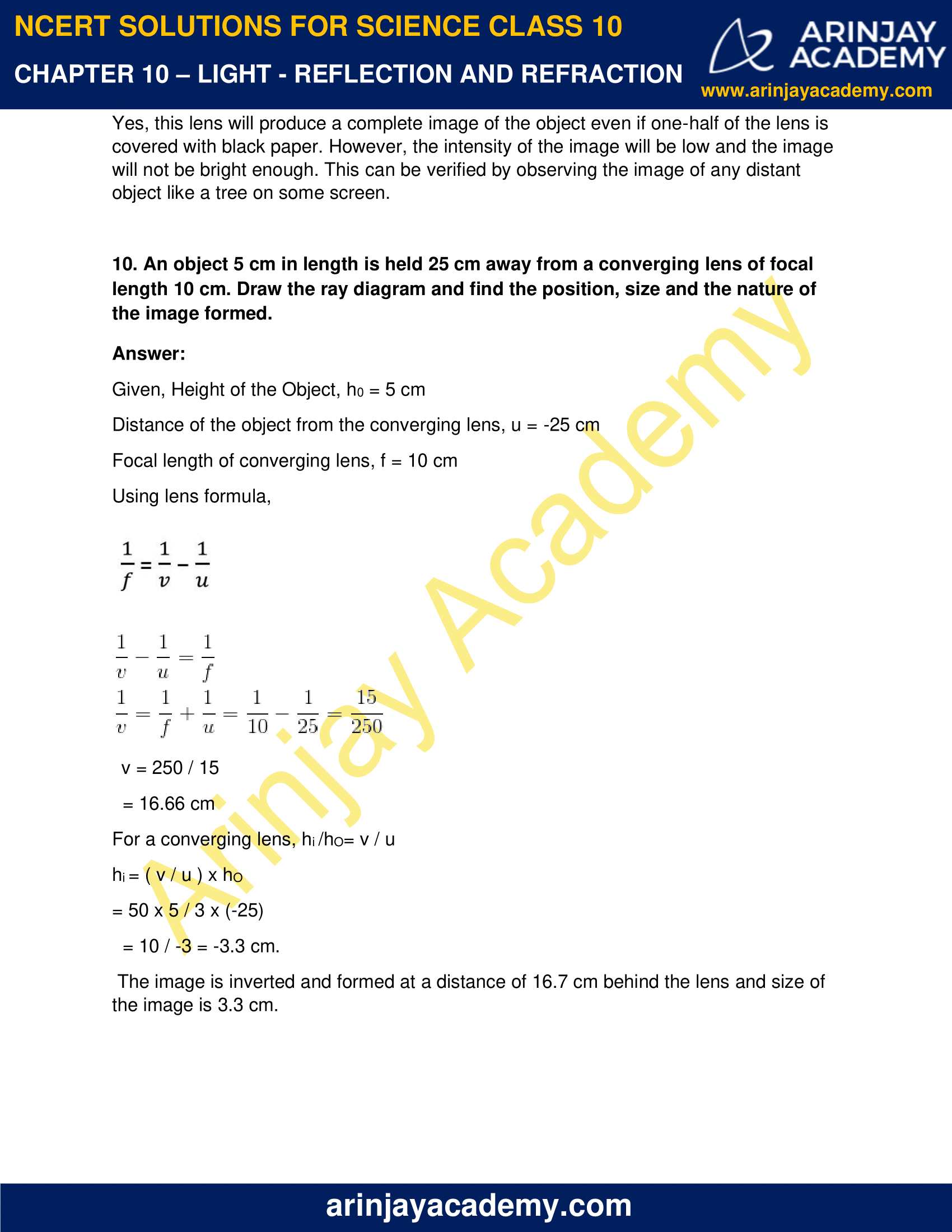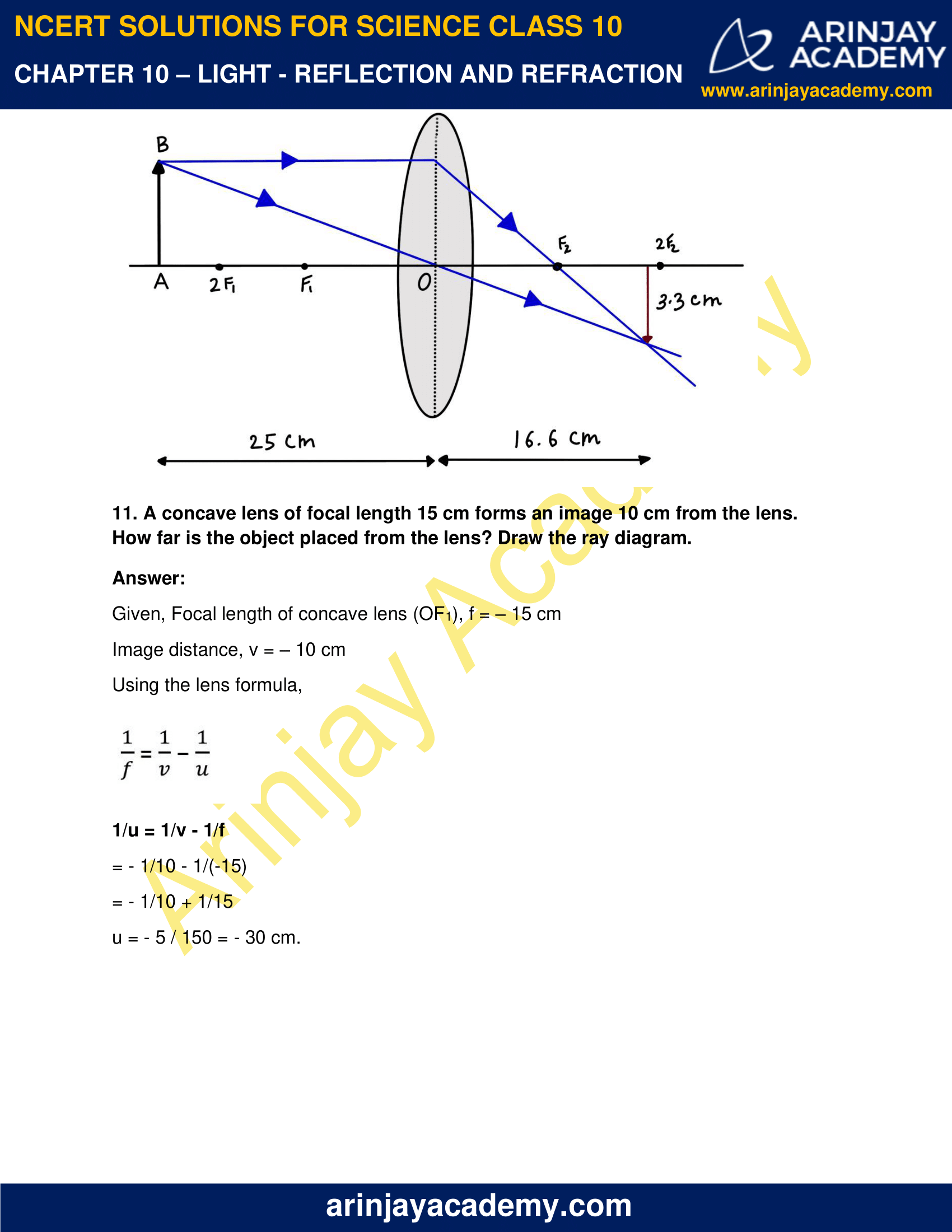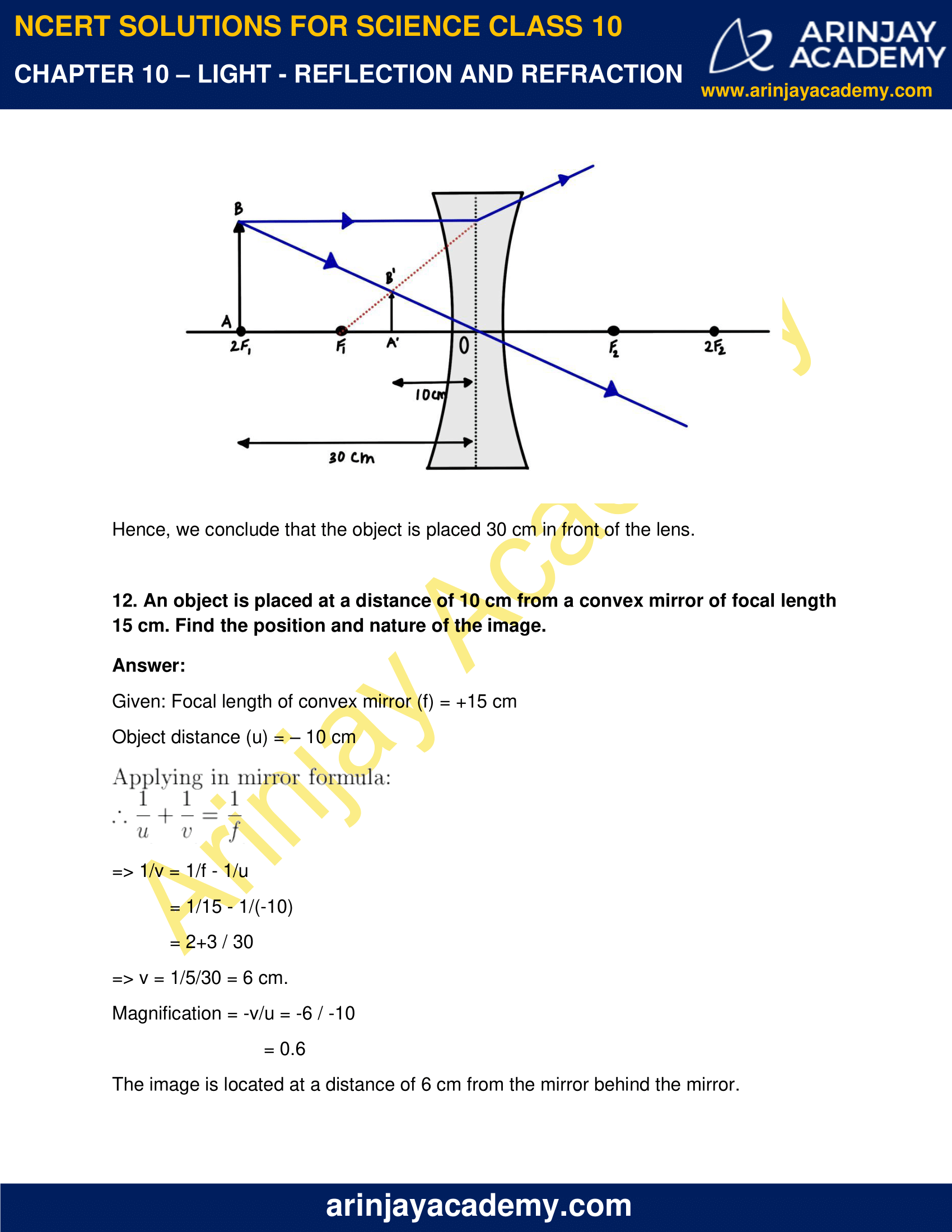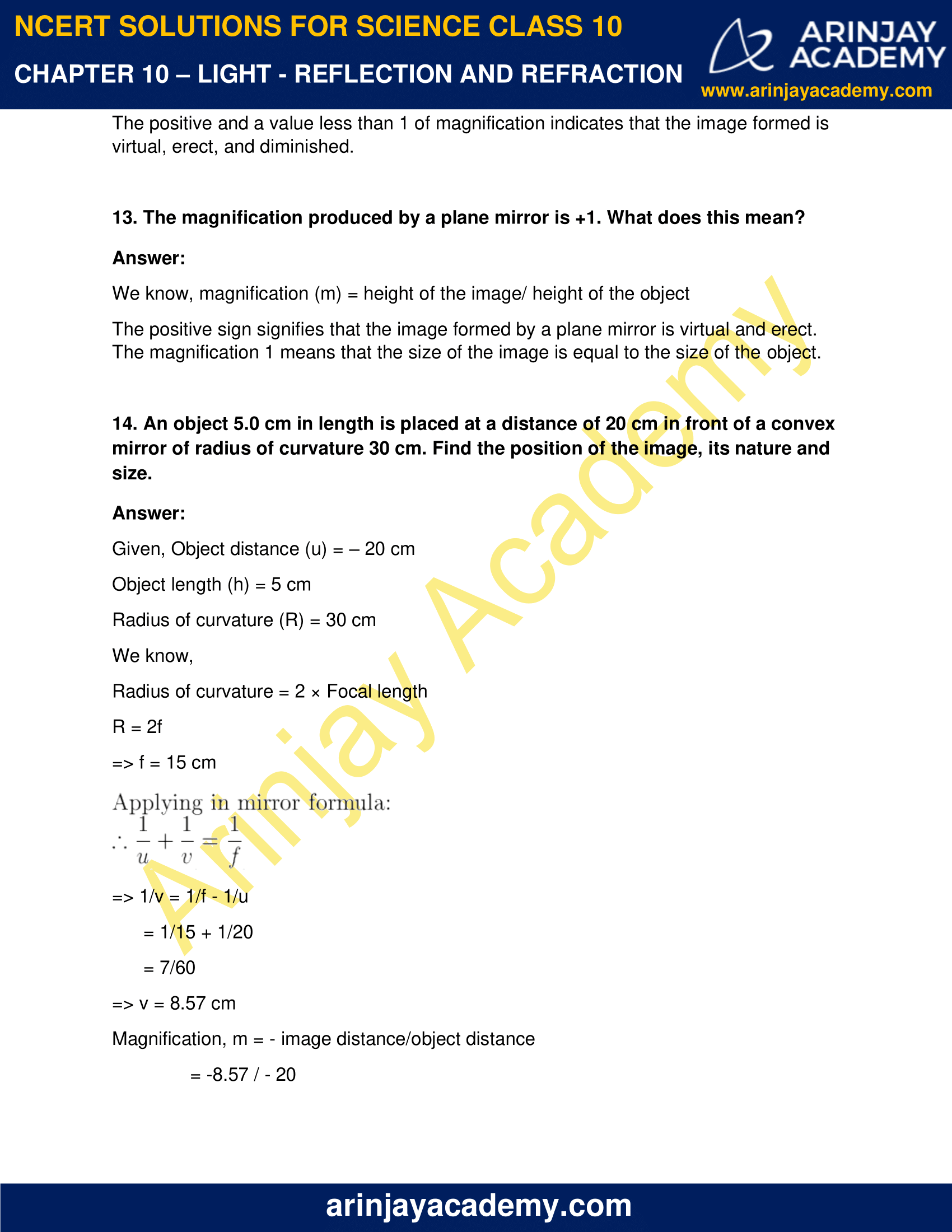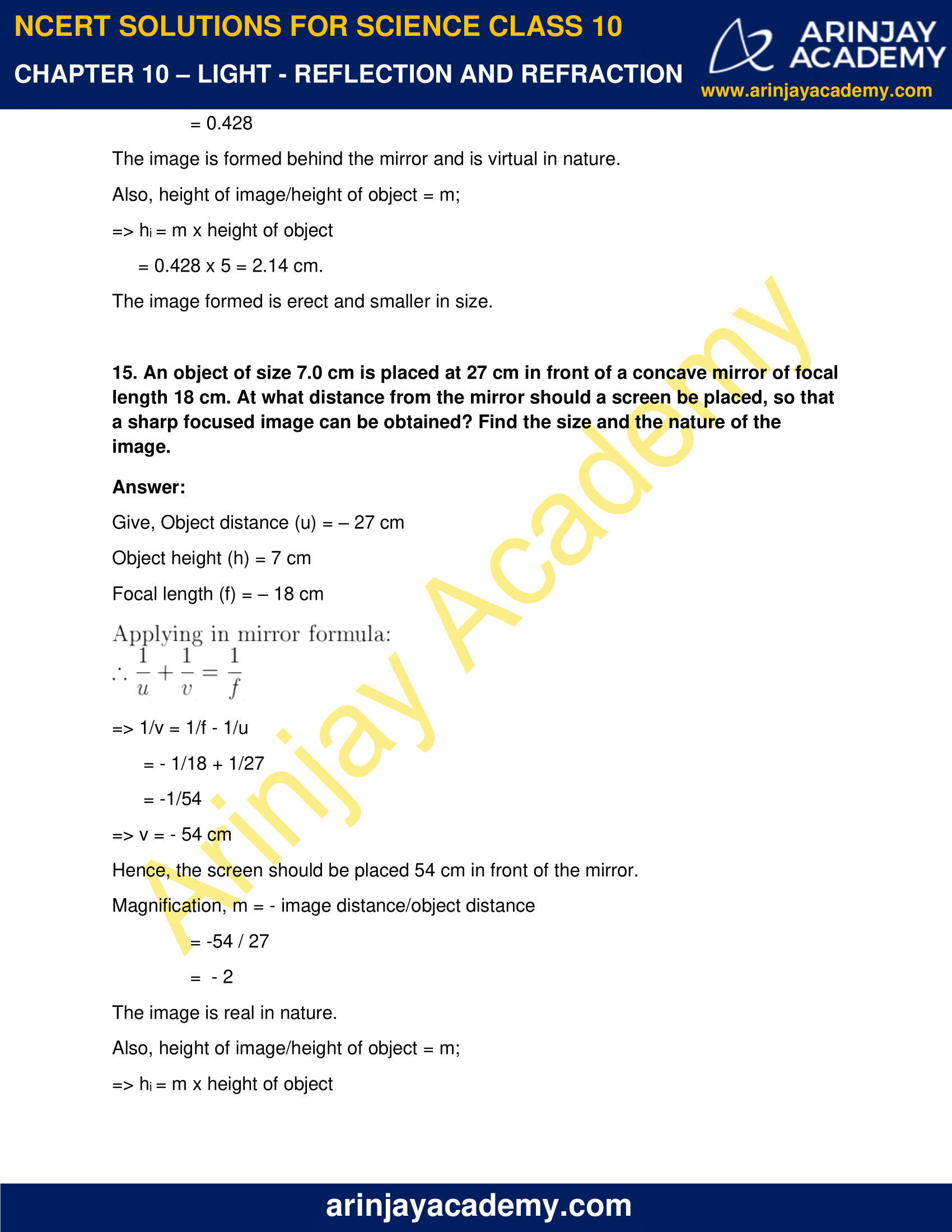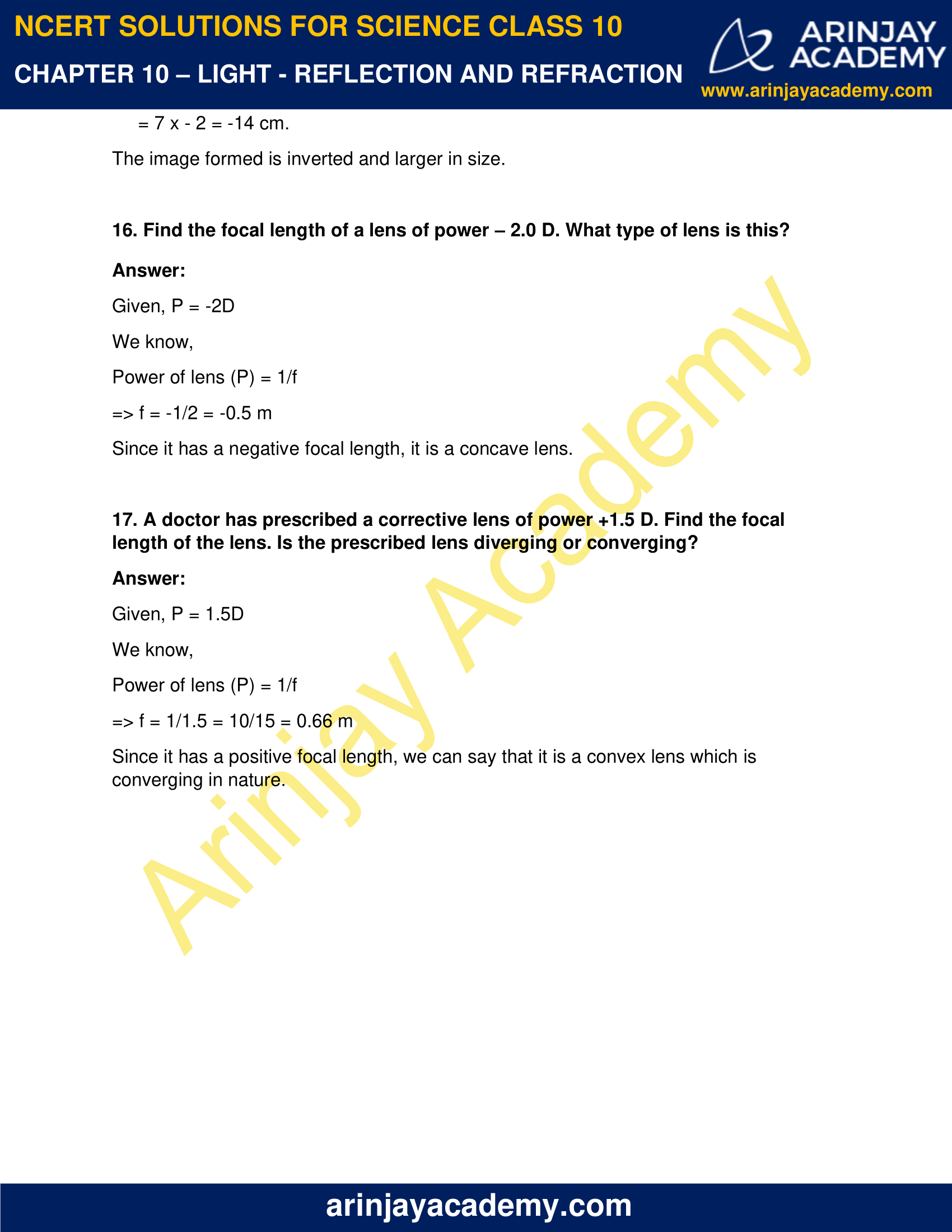### NCERT Solutions for Class 10 Science Chapter 10 – Light – Reflection and Refraction – NCERT Exercises

NCERT Solutions for Class 10 Science Chapter 10 – Light – Reflection and Refraction, Exercises includes all in text and exercise solved questions which helps you to understand the topic covered in Chapter 10 Light – Reflection and Refraction Class 10, in a better manner to help you to score good marks in your examinations.

### NCERT Solutions for Class 10 Science Chapter 10 – Intext Questions – Page 168

1. Define the principal focus of a concave mirror.

Light rays that are parallel to the principal axis of a concave mirror converge at a specific point on its principal axis after reflecting from the mirror. This point is called the principal focus of the concave mirror.

2. The radius of curvature of a spherical mirror is 20 cm. What is its focal length?

Given: Radius of curvature ( R ) = 20 cm

We know, Radius of curvature of a spherical mirror = 2 x Focal length

So, Focal length = R/2

= 20/2

= 10 cm.

Hence, the focal length of the given spherical mirror is 10 cm.

3. Name a mirror that can give an erect and enlarged image of an object.

Concave mirror gives an erect and enlarged image of an object when the object is placed between the pole and the principle focus.

4. Why do we prefer a convex mirror as a rear-view mirror in vehicles?

Convex mirror forms a virtual, erect, and diminished image of the object placed in front of it. We prefer a convex mirror as a rear-view mirror in vehicles to have a wider view of the road. It helps in assessing the traffic better.

### Intext Questions – Page 171

1. Find the focal length of a convex mirror whose radius of curvature is 32 cm.

Given: Radius of curvature (R) = 32 cm

We know, Radius of curvature = 2 × Focal length (f)

R = 2f

f= R/2

= 32/2 = 16 cm

Hence, the focal length of the given convex mirror is 16 cm.

2. A concave mirror produces three times magnified (enlarged) real image of an object placed at 10 cm in front of it. Where is the image located?

The relation between magnification, height of images, and image distances can be given by:

m = height of the image/ height of the object = – image distance/object distance

m = h1/h0  = – v / u

Let height of the object h0 = h

Then the height of the image h1 will be = – 3h (real image is formed)

On putting in equation and comparing:

– 3h / h = – v / u

v / u = 3

Image distance v = 3 X (- 10) = – 30 cm.

An inverted image is formed in front of the given concave mirror at a distance of 30 cm.

### Intext Questions – Page 176

1. A ray of light travelling in air enters obliquely into water. Does the light ray bend towards the normal or away from the normal? Why?

When a light ray enters from an optically rare medium (with lower refractive index) to an optically denser medium (with a higher refractive index), its speed slows down and bends towards the normal. Since water is denser than air, the ray will bend towards the normal.

2. Light enters from air to glass having refractive index 1.50. What is the speed of light in the glass? The speed of light in vacuum is 3 × 108 m s–1 .

Refractive index of a medium (nm) = Speed of light in vacuum/Speed of light in the medium

We know, Speed of light in vacuum (c) = 3 × 108 m/s

Given: Refractive index of glass (ng) = 1.50

So, Speed of light in the glass (v) = Speed of light in vacuum/ Refractive index of glass

= c/ng

=3 x 108/1.50

= 2x 108 ms-1

Hence, speed of light in glass is 2x 108 ms-1.

3. Find out, from Table 10.3, the medium having highest optical density. Also find the medium with lowest optical density.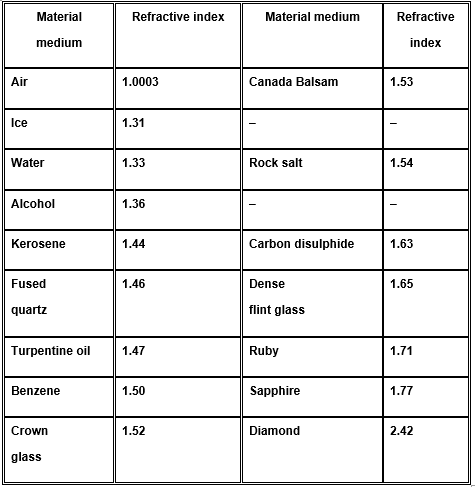A medium with the highest refractive index will have the highest optical density and vice-versa.

On the given basis:

Lowest Optical Density: Air with lowest refractive index of 1. 0003.

Highest Optical Density: Diamond with refractive index of 2.42.

4. You are given kerosene, turpentine and water. In which of these does the light travel fastest? Use the information given in Table 10.3.

Light will travel fastest in water as compared to kerosene & turpentine.

The speed of light is inversely proportional to the refractive index. Since according to the given table, the refractive index of water is lower than that of kerosene and turpentine, light will travel fastest in water.

5. The refractive index of diamond is 2.42. What is the meaning of this statement?

We know,

Refractive index of a medium (nm) = Speed of light in vacuum/Speed of light in the medium.

Diamond has a refractive index of 2.42.

Using the relation, Speed of light in medium = Speed of light in vacuum / 2.42

This shows that the speed of light in diamond will reduce by a factor of 2.42 as compared to its speed in the vacuum.

### NCERT Solutions for Class 10 Science Chapter 10 – Intext Questions – Page 184

1. Define 1 dioptre of power of a lens.

Power of a lens is the reciprocal of its focal length. Dioptre is the SI unit of power of the lens.

P = 1 / f (in m)

Hence, 1 dioptre can be defined as the power of a lens with focal length 1 metre.

2. A convex lens forms a real and inverted image of a needle at a distance of 50 cm from it. Where is the needle placed in front of the convex lens if the image is equal to the size of the object? Also, find the power of the lens.

For the image to be equal in the size of the object, the position of the image should be 2F.

Given: Object distance (u) = – 50 cm.

So, image distance (v) = 50 cm.

Let focal length = f

Focal length formula =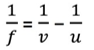1 / f = 1 / 50 – 1 / (-50)

= 1 / 50 + 1/ 50

=1 / 25

So, f = 25 cm = 0.25 m

Power, P = 1 / f

P = 1 / 0.25

P = +4D

Hence, power of lens is +4D.

3. Find the power of a concave lens of focal length 2 m.

Focal length of concave lens (f) = 2 m

Power of lens (P) = 1/f

= 1/ (-2)

= -0.5D.

Hence, the power of the given lens is -0.5D.

### NCERT Solutions for Class 10 Science Chapter 10 – NCERT Exercise – Page 185

1. Which one of the following materials cannot be used to make a lens? (a) Water (b) Glass (c) Plastic (d) Clay

(d) Clay; as it is opaque and does not allow the light to pass through it.

2. The image formed by a concave mirror is observed to be virtual, erect and larger than the object. Where should be the position of the object?

(a) Between the principal focus and the centre of curvature

(b) At the centre of curvature

(c) Beyond the centre of curvature

(d) Between the pole of the mirror and its principal focus.

(d) Between the pole of the mirror and its principal focus.

3. Where should an object be placed in front of a convex lens to get a real image of the size of the object?

(a) At the principal focus of the lens

(b) At twice the focal length

(c) At infinity

(d) Between the optical centre of the lens and its principal focus.

(b) At twice the focal length

4. A spherical mirror and a thin spherical lens have each a focal length of –15 cm. The mirror and the lens are likely to be

(a) both concave.

(b) both convex.

(c) the mirror is concave and the lens is convex.

(d) the mirror is convex, but the lens is concave.

(a) Both concave

5. No matter how far you stand from a mirror, your image appears erect. The mirror is likely to be

(a) only plane

(b) only concave

(c) only convex

(d) either plane or convex

(d) Either plane or convex

6. Which of the following lenses would you prefer to use while reading small letters found in a dictionary?

(a) A convex lens of focal length 50 cm.

(b) A concave lens of focal length 50 cm.

(c) A convex lens of focal length 5 cm.

(d) A concave lens of focal length 5 cm.

(c ) A convex lens of focal length 5 cm; When object is placed between radius of curvature and focal length.

7. We wish to obtain an erect image of an object, using a concave mirror of focal length 15 cm. What should be the range of distance of the object from the mirror? What is the nature of the image? Is the image larger or smaller than the object? Draw a ray diagram to show the image formation in this case.

In a concave mirror, to obtain an erect image, we need to put the object between the pole and the focus. So, the range of the object from the mirror should be between 0 cm to 15 cm.

The image will be erect, virtual, and magnified.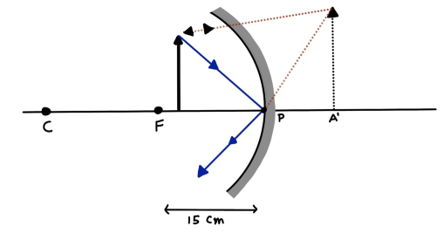8. Name the type of mirror used in the following situations.

(b) Side/rear-view mirror of a vehicle.

(c) Solar furnace

(a) Concave Mirror is used in the headlights of a car. Concave mirrors can produce powerful parallel beams of light when light source is placed on their principal focus.

(b) Convex mirror is used in side/rear-view of a vehicle because it makes a virtual, erect, and diminished image. This helps in getting a wider view of the traffic behind the vehicle.

(c) Concave Mirror is used in solar furnace because it concentrates the parallel rays of sun at principal focus making it possible to concentrate heat a point.

9. One-half of a convex lens is covered with a black paper. Will this lens produce a complete image of the object? Verify your answer experimentally. Explain your observations.

Yes, this lens will produce a complete image of the object even if one-half of the lens is covered with black paper. However, the intensity of the image will be low and the image will not be bright enough. This can be verified by observing the image of any distant object like a tree on some screen.

10. An object 5 cm in length is held 25 cm away from a converging lens of focal length 10 cm. Draw the ray diagram and find the position, size and the nature of the image formed.

Given, Height of the Object, h0 = 5 cm

Distance of the object from the converging lens, u = -25 cm

Focal length of converging lens, f = 10 cm

Using lens formula,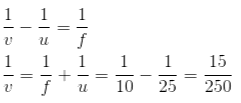v = 250 / 15

= 16.66 cm

For a converging lens, hi /hO= v / u

hi = ( v / u ) x hO

= 50 x 5 / 3 x (-25)

= 10 / -3 = -3.3 cm.

The image is inverted and formed at a distance of 16.7 cm behind the lens and size of the image is 3.3 cm.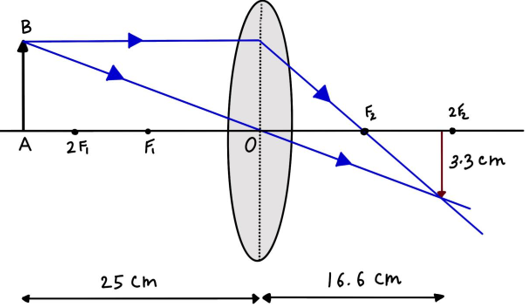11. A concave lens of focal length 15 cm forms an image 10 cm from the lens. How far is the object placed from the lens? Draw the ray diagram.

Given, Focal length of concave lens (OF1), f = – 15 cm

Image distance, v = – 10 cm

Using the lens formula,1/u = 1/v – 1/f

= – 1/10 – 1/(-15)

= – 1/10 + 1/15

u = – 5 / 150 = – 30 cm.

Hence, we conclude that the object is placed 30 cm in front of the lens.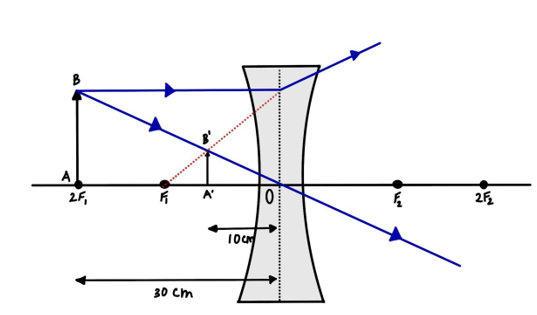12. An object is placed at a distance of 10 cm from a convex mirror of focal length 15 cm. Find the position and nature of the image.

Given: Focal length of convex mirror (f) = +15 cm

Object distance (u) = – 10 cm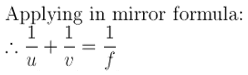=> 1/v = 1/f – 1/u

= 1/15 – 1/(-10)

= 2+3 / 30

=> v = 1/5/30 = 6 cm.

Magnification = -v/u = -6 / -10

= 0.6

The image is located at a distance of 6 cm from the mirror behind the mirror.

The positive and a value less than 1 of magnification indicates that the image formed is virtual, erect, and diminished.

13. The magnification produced by a plane mirror is +1. What does this mean?

We know, magnification (m) = height of the image/ height of the object

The positive sign signifies that the image formed by a plane mirror is virtual and erect. The magnification 1 means that the size of the image is equal to the size of the object.

14. An object 5.0 cm in length is placed at a distance of 20 cm in front of a convex mirror of radius of curvature 30 cm. Find the position of the image, its nature and size.

Given, Object distance (u) = – 20 cm

Object length (h) = 5 cm

Radius of curvature (R) = 30 cm

We know,

Radius of curvature = 2 × Focal length

R = 2f

=> f = 15 cm

=> 1/v = 1/f – 1/u

= 1/15 + 1/20

= 7/60

=> v = 8.57 cm

Magnification, m = – image distance/object distance

= -8.57 / – 20

= 0.428

The image is formed behind the mirror and is virtual in nature.

Also, height of image/height of object = m;

=> hi = m x height of object

= 0.428 x 5 = 2.14 cm.

The image formed is erect and smaller in size.

15. An object of size 7.0 cm is placed at 27 cm in front of a concave mirror of focal length 18 cm. At what distance from the mirror should a screen be placed, so that a sharp focused image can be obtained? Find the size and the nature of the image.

Give, Object distance (u) = – 27 cm

Object height (h) = 7 cm

Focal length (f) = – 18 cm=> 1/v = 1/f – 1/u

= – 1/18 + 1/27

= -1/54

=> v = – 54 cm

Hence, the screen should be placed 54 cm in front of the mirror.

Magnification, m = – image distance/object distance

= -54 / 27

=  – 2

The image is real in nature.

Also, height of image/height of object = m;

=> hi = m x height of object

= 7 x – 2 = -14 cm.

The image formed is inverted and larger in size.

16. Find the focal length of a lens of power – 2.0 D. What type of lens is this?

Given, P = -2D

We know,

Power of lens (P) = 1/f

=> f = -1/2 = -0.5 m

Since it has a negative focal length, it is a concave lens.

17. A doctor has prescribed a corrective lens of power +1.5 D. Find the focal length of the lens. Is the prescribed lens diverging or converging?

Given, P = 1.5D

We know,

Power of lens (P) = 1/f

=> f = 1/1.5 = 10/15 = 0.66 m

Since it has a positive focal length, we can say that it is a convex lens which is converging in nature.

### Topics Covered in Light – Reflection and Refraction Class 10 Science

• Reflection
• Spherical Mirrors
• Image Formation by Spherical Mirrors
• Representation of Images Formed by Spherical Mirrors Using Ray Diagrams
• Sign Convention for Reflection by Spherical Mirrors
• Mirror Formula and Magnification
• Refraction of Light
• Refraction through a Rectangular Glass Slab
• The Refractive Index
• Refraction by Spherical Lenses
• Image Formation by Lenses
• Sign Convention for Spherical Lenses
• Lens Formula and Magnification
• Power of a Lens